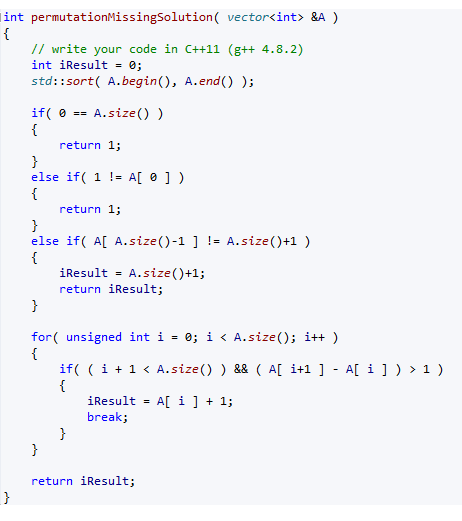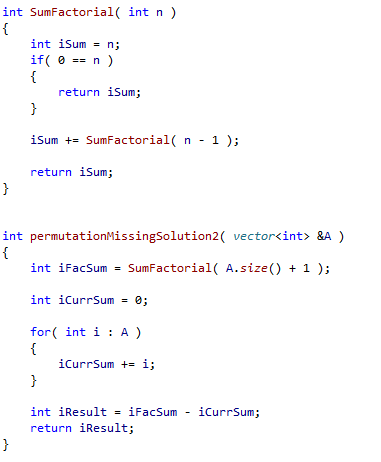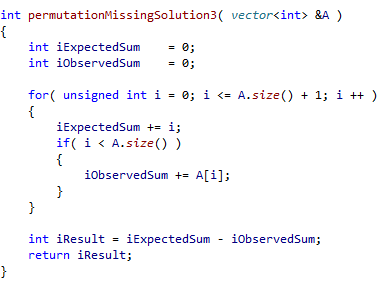A zero-indexed array A consisting of N different integers is given. The array contains integers in the range [1..(N + 1)], which means that exactly one element is missing.

Your goal is to find that missing element. So in [2,3,1,5] you should return 4.

This must be done in O(N) time. N can range from 0 to 100,000 (so guard against empty arrays). Each element in A is distinct and ranges from 1 to N+1.

Language used: C++

Solution 1
One solution is to sort the array and look for two numbers whose difference is larger than one.This means you can quickly interrogate whether 1 is the missing element, because it would be A, and the last number must be N+1. The approach requires you to explicitly ensure the array is not empty.

Solution 2: Recursion

If you really want you can use recursion to sum the numbers that you should have, and the difference between that and the numbers in A will give you the missing number.  At the time of writing Codility gave me 100% for this. However Visual Studio gave me a very upset Stack Overflow with my current stack parameters:Solution 3: Looping

Recursion can be a useful technique, and this is a very simple exercise to try it with. However it is completely unneccessary here. We can just use a loop to sum the ints in the array and what we would expect to have, then take the difference as our answer: